Next: Hydrostatic Equilibrium Up: Magnetohydrodynamics Previous: Basic Equations of Ideal   Contents

## Axisymmetric Case

The basic equations to be solved are the magnetohydrodynamical equations and the Poisson equation for the gravitational potential. In cylindrical coordinates (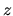,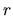,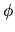) with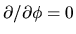, the equations are expressed as follows: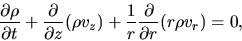(B.11)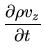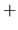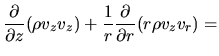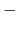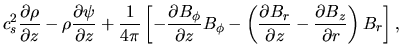(B.12)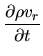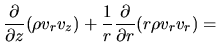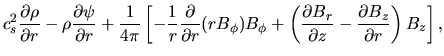(B.13)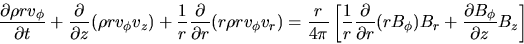(B.14)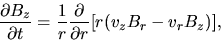(B.15)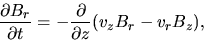(B.16)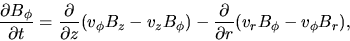(B.17)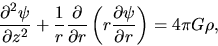(B.18)

where the variables have their ordinary meanings. Equation (B.11) is the continuity equation; equations (B.12), (B.13) and (B.14) are the equations of motion. The induction equations for the poloidal magnetic fields are equations (B.15) and (B.16) and for the toroidal magnetic field is equation (B.17). The last equation (B.18) is the Poisson equation.Next: Hydrostatic Equilibrium Up: Magnetohydrodynamics Previous: Basic Equations of Ideal   Contents
Kohji Tomisaka 2007-07-08